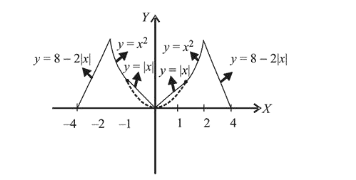# Let f(x)

Question:

Let $f(x)=\left\{\begin{array}{cc}\max \left\{|x|, x^{2}\right\} & |x| \leq 2 \\ 8-2|x|, & 2<|x| \leq 4\end{array}\right.$

Let $\mathrm{S}$ be the set of points in the interval $(-4,4)$ at which $f$ is not differentiable. Then $\mathrm{S}$ :

1. (1) is an empty set

2. (2) equals $\{-2,-1,0,1,2\}$

3. (3) equals $\{-2,-1,1,2\}$

4. (4) equals $\{-2,2\}$

Correct Option: , 2

Solution:

Given $f(x)=\left\{\begin{array}{cc}\max \left\{|x|, x^{2}\right\} & |x| \leq 2 \\ 8-2|x| & 2<|\mathrm{x}| \leq 4\end{array}\right.$$\because f(x)$ is not differentiable at $-2,-1,0,1$ and 2 .

$\therefore S=\{-2,-1,0,1,2\}$